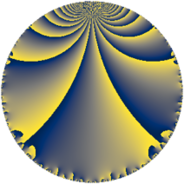# Properties

 Label 63.4.pLevel $63$ Weight $4$ Character orbit 63.p Rep. character $\chi_{63}(17,\cdot)$ Character field $\Q(\zeta_{6})$ Dimension $16$ Newform subspaces $1$ Sturm bound $32$ Trace bound $0$

# Related objects

## Defining parameters

 Level: $$N$$ $$=$$ $$63 = 3^{2} \cdot 7$$ Weight: $$k$$ $$=$$ $$4$$ Character orbit: $$[\chi]$$ $$=$$ 63.p (of order $$6$$ and degree $$2$$) Character conductor: $$\operatorname{cond}(\chi)$$ $$=$$ $$21$$ Character field: $$\Q(\zeta_{6})$$ Newform subspaces: $$1$$ Sturm bound: $$32$$ Trace bound: $$0$$

## Dimensions

The following table gives the dimensions of various subspaces of $$M_{4}(63, [\chi])$$.

Total New Old
Modular forms 56 16 40
Cusp forms 40 16 24
Eisenstein series 16 0 16

## Trace form

 $$16q + 32q^{4} + 56q^{7} + O(q^{10})$$ $$16q + 32q^{4} + 56q^{7} - 72q^{10} - 188q^{16} - 612q^{19} + 528q^{22} - 20q^{25} + 1036q^{28} + 1128q^{31} - 1196q^{37} - 3204q^{40} + 328q^{43} - 1392q^{46} + 784q^{49} + 4452q^{52} - 3372q^{58} - 1632q^{61} + 5432q^{64} + 308q^{67} + 3612q^{70} + 4068q^{73} - 2176q^{79} - 10188q^{82} - 4608q^{85} + 708q^{88} + 924q^{91} - 2916q^{94} + O(q^{100})$$

## Decomposition of $$S_{4}^{\mathrm{new}}(63, [\chi])$$ into newform subspaces

Label Dim. $$A$$ Field CM Traces $q$-expansion
$$a_2$$ $$a_3$$ $$a_5$$ $$a_7$$
63.4.p.a $$16$$ $$3.717$$ $$\mathbb{Q}[x]/(x^{16} - \cdots)$$ None $$0$$ $$0$$ $$0$$ $$56$$ $$q+\beta _{1}q^{2}+(4+\beta _{2}-4\beta _{3}+\beta _{9})q^{4}+\cdots$$

## Decomposition of $$S_{4}^{\mathrm{old}}(63, [\chi])$$ into lower level spaces

$$S_{4}^{\mathrm{old}}(63, [\chi]) \cong$$ $$S_{4}^{\mathrm{new}}(21, [\chi])$$$$^{\oplus 2}$$